## Physical Intuition

In the previous lecture we mentioned a relation between velocity, distance, and the meaning of integration, which gave you a physical way of thinking about integration. In this section we generalize our previous observation.

The following is a restatement of the fundamental theorem of calculus:

Theorem 2.2.6 (Net Change Theorem)   The definite integral of the rate of change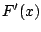of some quantity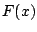is the net change in that quantity: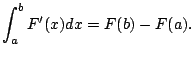For example, if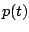is the population of students at UCSD at time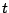, then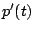is the rate of change. Latelyhas been positive sinceis growing (rapidly!). The net change interpretation of integration is that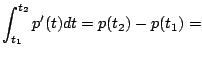change in number of students from time $t_1$ to $t_2$Another very common example you'll seen in problems involves water flow into or out of something. If the volume of water in your bathtub is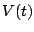gallons at time(in seconds), then the rate at which your tub is draining is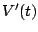gallons per second. If you have the geekiest drain imaginable, it prints out the drainage rate. You can use that printout to determine how much water drained out from time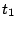to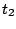: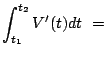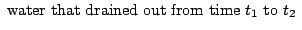Some problems will try to confuse you with different notions of change. A standard example is that if a car has velocity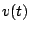, and you drive forward, then slam it in reverse and drive backward to where you start (say 10 seconds total elapse), thenis positive some of the time and negative some of the time. The integral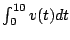is not the total distance registered on your odometer, sinceis partly positive and partly negative. If you want to express how far you actually drove going back and forth, compute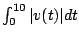. The following example emphasizes this distinction:

Example 2.2.7   An ancient dragon is pacing on the cliffs in Del Mar, and has velocity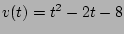. Find (1) the displacement of the dragon from time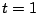until time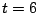(i.e., how far the dragon is at time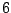from where it was at time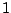), and (2) the total distance the dragon paced from timeto.

For (1), we compute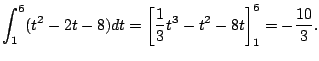For (2), we compute the integral of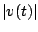: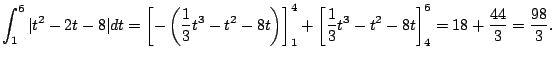William Stein 2006-03-15